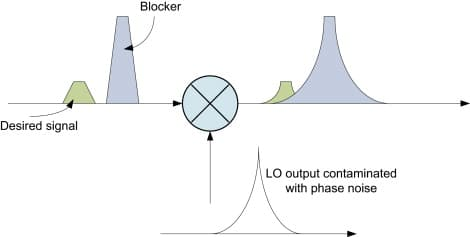# 混频器的干扰

\begin{align*} \newcommand{\dif}{\mathop{}\!\mathrm{d}} \newcommand{\p}{\partial} \end{align*}

# 组合频率干扰

$f_k=\pm pf_0\pm qf_s\approx f_i\\ p,q\in\mathbb{Z^+}$

$f_i\approx \begin{cases} pf_0-qf_s\\ -pf_0+qf_s \end{cases}\\ 可得：f_s\approx\frac{p\pm 1}{q-p} f_i$

$f_i\approx \begin{cases} pf_0-qf_n\\ -pf_0+qf_n \end{cases}\\ 可得：f_n\approx\frac{1}{q}(pf_0\pm f_i)\\ f_n\approx\frac{1}{q}[pf_s+(p\pm1)f_i]$

# 副波道干扰

1. 当噪声信号 $f_n$ 接近于中频信号 $f_i=f_0-f_s$ 时，产生的 中频干扰（intermediate frequency interference），此时噪声会直接干扰 $f_i$
2. 当噪声信号 $f_n$ 接近于 $f=f_s+2f_i=f_0+f_i$ 时，产生的 镜像频率干扰（image frequency interference），此时噪声经过差拍 $f_n-f_0=f_i$ 也会产生对 $f_i$ 的干扰。

# 调制中的干扰

## 阻塞现象与相互混频# 克服干扰的措施

1. 提高前端电路（天线回路和高放）的选择性；
2. 合理选择中频，使组合频率远离中频（比如选高中频+二级混频）；
3. 合理选择电子器件与静态工作点；
4. 采用各种平衡电路（差分电路），使噪声相减后相消。